# The blue curves shown in the figure (Figure 1) display the motion of two different particles...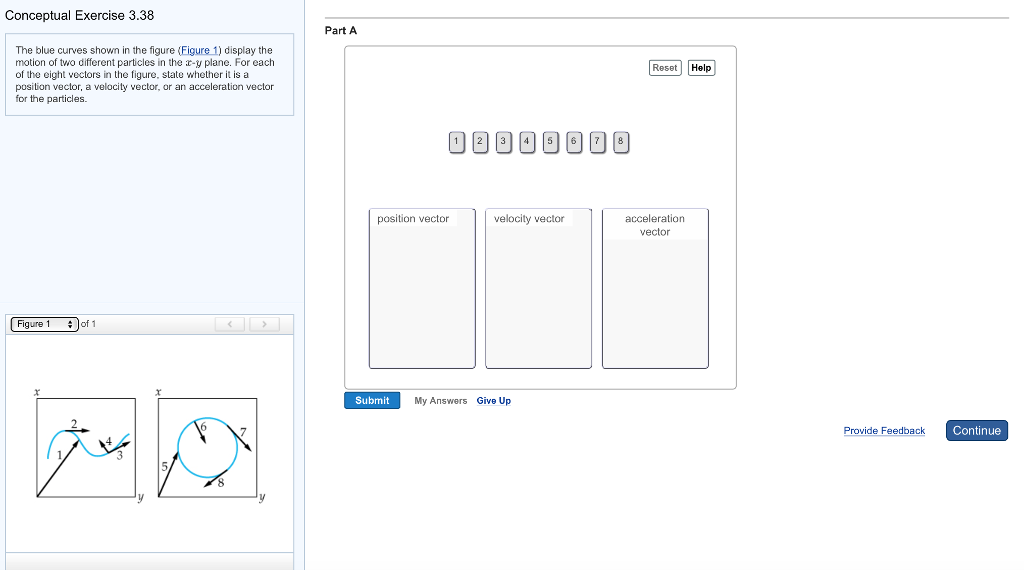The blue curves shown in the figure (Figure 1) display the motion of two different particles in the x-y plane. For each of the eight vectors in the figure, state whether it is a position vector, a velocity vector, or an acceleration vector for the particles.

Position vectors: 1, 5.

Velocity vectors: 2, 3, 7, 8.

Acceleration vectors: 4, 6.

#### Earn Coin

Coins can be redeemed for fabulous gifts.

Similar Homework Help Questions
• ### Shown in figure is a Scotch yoke mechanism. What is the input and output motion of...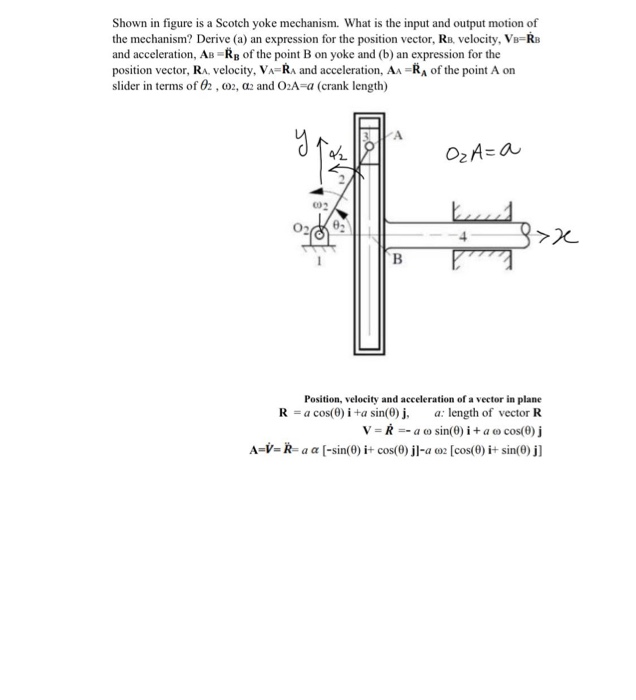Shown in figure is a Scotch yoke mechanism. What is the input and output motion of the mechanism? Derive (a) an expression for the position vector, Re, velocity, V=RB and acceleration, AB =Rg of the point B on yoke and (b) an expression for the position vector, RA, velocity, VA=RA and acceleration, AA =RA of the point A on slider in terms of 02 , 02, 2 and 02A=a (crank length) y O2A=a 002 I >> B Position, velocity and...

• ### Shown in figure is a Scotch yoke mechanism. What is the input and output motion of...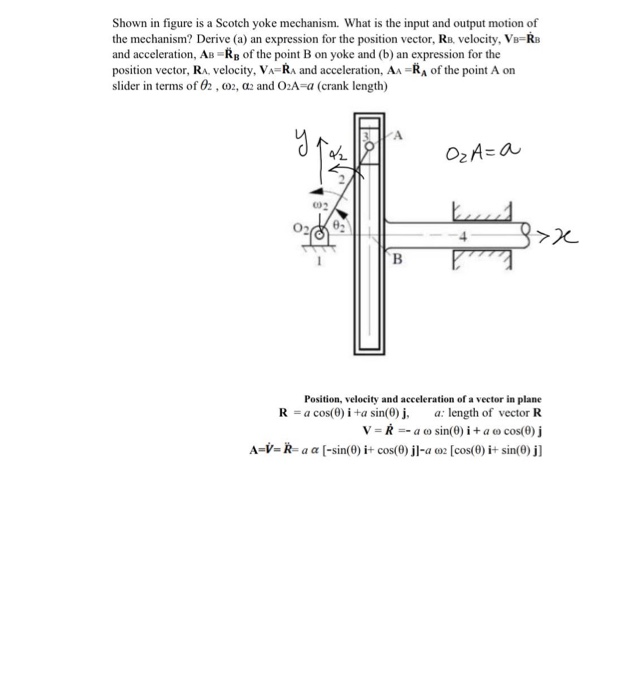Shown in figure is a Scotch yoke mechanism. What is the input and output motion of the mechanism? Derive (a) an expression for the position vector, Re, velocity, V=RB and acceleration, AB =Rg of the point B on yoke and (b) an expression for the position vector, RA, velocity, VA=RA and acceleration, AA =RA of the point A on slider in terms of 02, 02, 2 and 02A=a (crank length) Α. y OzA=a 002 I >> B Position, velocity and...

• ### Shown in figure is a Scotch yoke mechanism. What is the input and output motion of...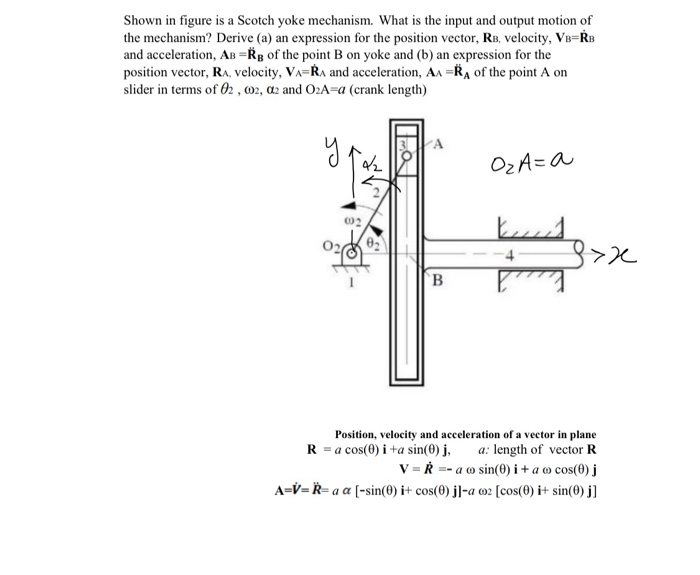Shown in figure is a Scotch yoke mechanism. What is the input and output motion of the mechanism? Derive (a) an expression for the position vector, Re, velocity, Vo=RB and acceleration, AB =Rp of the point B on yoke and (b) an expression for the position vector, RA, velocity, VARA and acceleration, AA =RA of the point A on slider in terms of 02, 02, d2 and O2A=a (crank length) y A 42 OzA=a 002 Ix B Position, velocity and...

• ### What quantities are shown on a complete motion diagram?Please help, I can pick only one of them asan answer, I guess i thought of it wrongWhat quantities are shown on a completemotion diagram?Only the position of the object in each frameof the film, shown as a dotOnly the average velocity vectors (found byconnecting each dot in the motion diagram to the next with a vectorarrow)Only the average acceleration vectors (whereeach acceleration vector links every two velocity vectors)All of the aboveTry Again

• ### Part B Review The following figure shows the first three points of a motion diagram.(Figure 1)...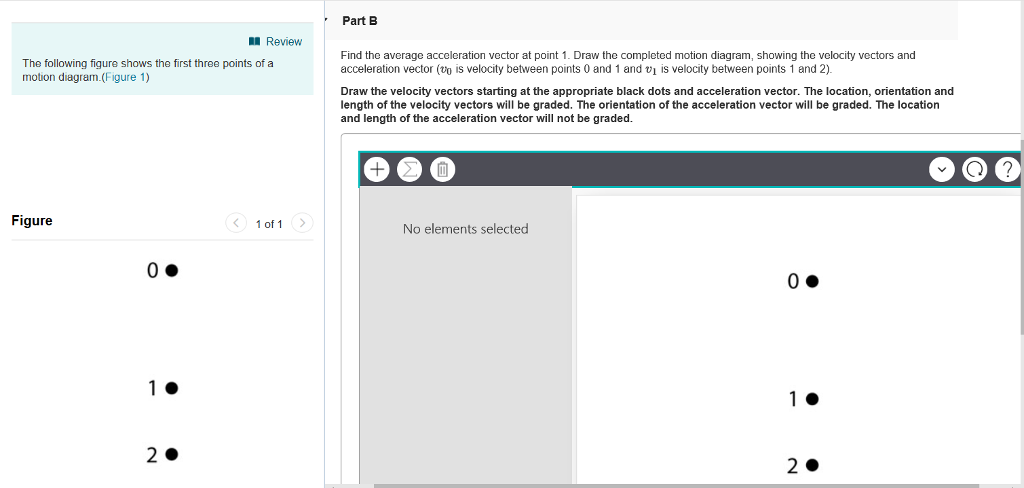Part B Review The following figure shows the first three points of a motion diagram.(Figure 1) Find the average acceleration vector at point 1. Draw the completed motion diagram, showing the velocity vectors and acceleration vector (v is velocity between points 0 and 1 and v is velocity between points 1 and 2) Draw the velocity vectors starting at the appropriate black dots and acceleration vector. The location, orientation and length of the velocity vectors will be graded. The orientation...

• ### 7. Do the projectile motion General Motion with Unit Vectors and Components An object undergoes the...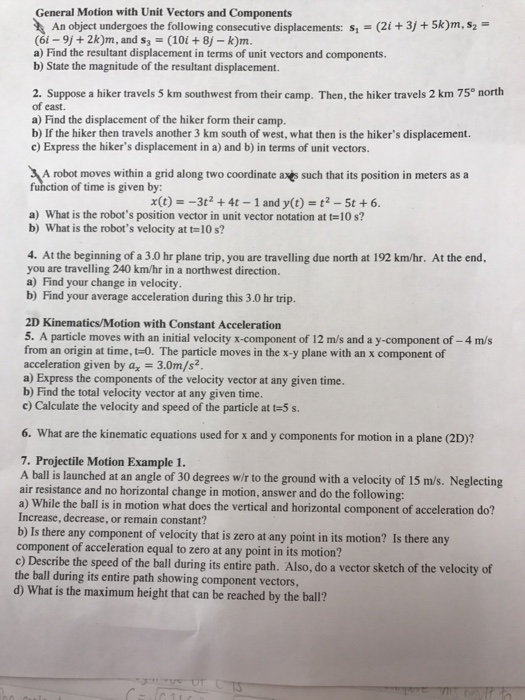7. Do the projectile motion General Motion with Unit Vectors and Components An object undergoes the following consecutive displacements: s (2i +3j +5k)m,s2 (6i- 9j + 2k)m, and s3 (10i 8j -k)m. a) Find the resultant displacement in terms of unit vectors and components. b) State the magnitude of the resultant displacement. 2. Suppose a hiker travels 5 km southwest from their camp. Then, the hiker travels 2 km 75° north of east. a) Find the displacement of the hiker...

• ### 7. General Motion with Unit Vectors and Components An object undergoes the following consecutive displacements: s...7. General Motion with Unit Vectors and Components An object undergoes the following consecutive displacements: s (2i +3j +5k)m,s2 (6i- 9j + 2k)m, and s3 (10i 8j -k)m. a) Find the resultant displacement in terms of unit vectors and components. b) State the magnitude of the resultant displacement. 2. Suppose a hiker travels 5 km southwest from their camp. Then, the hiker travels 2 km 75° north of east. a) Find the displacement of the hiker form their camp. b)...

• ### 4. General Motion with Unit Vectors and Components An object undergoes the following consecutive displacements: s...4. General Motion with Unit Vectors and Components An object undergoes the following consecutive displacements: s (2i +3j +5k)m,s2 (6i- 9j + 2k)m, and s3 (10i 8j -k)m. a) Find the resultant displacement in terms of unit vectors and components. b) State the magnitude of the resultant displacement. 2. Suppose a hiker travels 5 km southwest from their camp. Then, the hiker travels 2 km 75° north of east. a) Find the displacement of the hiker form their camp. b)...

• ### 5. General Motion with Unit Vectors and Components An object undergoes the following consecutive displacements: s...5. General Motion with Unit Vectors and Components An object undergoes the following consecutive displacements: s (2i +3j +5k)m,s2 (6i- 9j + 2k)m, and s3 (10i 8j -k)m. a) Find the resultant displacement in terms of unit vectors and components. b) State the magnitude of the resultant displacement. 2. Suppose a hiker travels 5 km southwest from their camp. Then, the hiker travels 2 km 75° north of east. a) Find the displacement of the hiker form their camp. b)...

• ### 2. General Motion with Unit Vectors and Components An object undergoes the following consecutive displacements: s...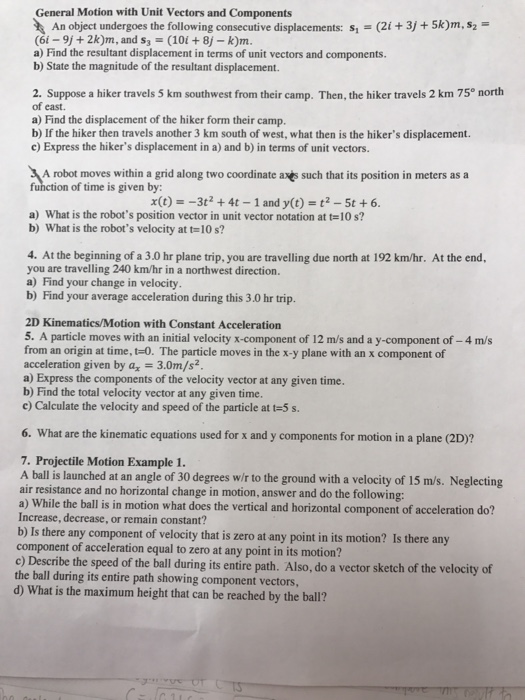2. General Motion with Unit Vectors and Components An object undergoes the following consecutive displacements: s (2i +3j +5k)m,s2 (6i-91+ 2krn, and s,-(10i + 8j-km a) Find the resultant displacement in terms of unit vectors and components. b) State the magnitude of the resultant displacement. 2. Suppose a hiker travels 5 km southwest from their camp. Then, the hiker travels 2 km 75° north of east. a) Find the displacement of the hiker form their camp. b) If the hiker...